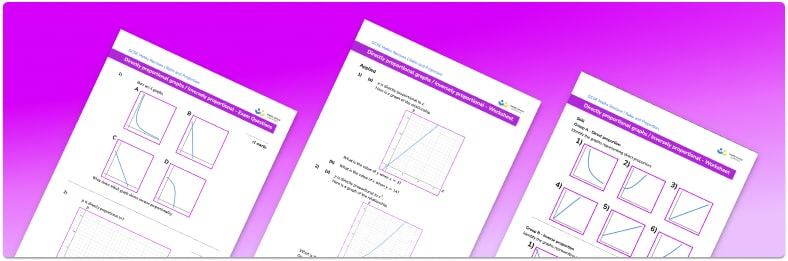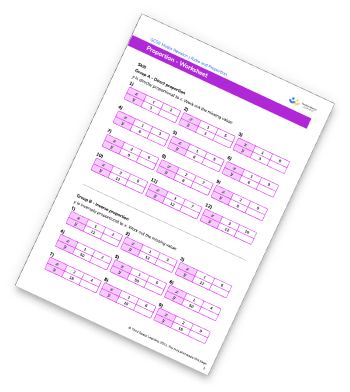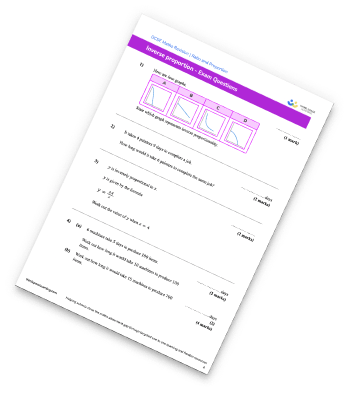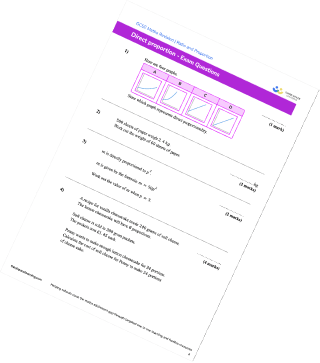# Directly Proportional Graph/ Inversely Proportional Graph Worksheet• This field is for validation purposes and should be left unchanged.

You can unsubscribe at any time (each email we send will contain an easy way to unsubscribe). To find out more about how we use your data, see our privacy policy.

Help your students prepare for their Maths GCSE with this free directly proportional graph/ Inversely proportional graph worksheet of 24 questions and answers

• Section 1 of the directly proportional graph/ Inversely proportional graph worksheet  contains 18 skills-based directly proportional graph/ Inversely proportional graph questions, in 3 groups to support differentiation
• Section 2 contains 3 applied directly proportional graph/ Inversely proportional graph questions with a mix of worded problems and deeper problem solving questions
• Section 3 contains 4 foundation and higher level GCSE exam style directly proportional graph/ Inversely proportional graph questions
• Answers and a mark scheme for all directly proportional graph/ Inversely proportional graph questions are provided
• Questions follow variation theory with plenty of opportunities for students to work independently at their own level
• All questions created by fully qualified expert secondary maths teachers
• Suitable for GCSE maths revision for AQA, OCR and Edexcel exam boards

### Directly proportional graph/ Inversely proportional graph at a glance

If two amounts are proportional then the ratio between them is always the same. There are two types of proportionality; direct or inverse (indirect). For direct proportion, as one amount increases so does the other, e.g. converting currency. For inverse proportion, as one amount increases the other decreases, e.g. the number of hours to complete a task assigned to multiple people.

We can draw graphs to represent proportional relationships. Graphs showing direct proportion will always pass through the origin. Their shape will depend on the nature of the relationship. If y is directly proportional to x then the graph will be a straight line. The gradient of the straight line is the rate of change, known as the constant of proportionality, k. A good real life example of this is a currency conversion graph, where the gradient is equal to the exchange rate.

If y is directly proportional to x2 then the graph will take the shape of a quadratic graph.

If y is inversely proportional to x, then y is proportional to 1 divided by x. The shape of a graph showing inverse proportion is that of a reciprocal graph.

We can read and find missing values from proportion graphs and use these to solve proportion problems.

Looking forward, students can then progress to additional ratio and proportion worksheets, for example a ratio worksheet or a ratio worksheet.

For more teaching and learning support on Ratio and Proportion our GCSE maths lessons provide step by step support for all GCSE maths concepts.

## Related worksheets

Proportion WorksheetInverse Proportion WorksheetDirect Proportion Worksheet## Do you have KS4 students who need more focused attention to succeed at GCSE?There will be students in your class who require individual attention to help them succeed in their maths GCSEs. In a class of 30, it’s not always easy to provide.

Help your students feel confident with exam-style questions and the strategies they’ll need to answer them correctly with our dedicated GCSE maths revision programme.

Lessons are selected to provide support where each student needs it most, and specially-trained GCSE maths tutors adapt the pitch and pace of each lesson. This ensures a personalised revision programme that raises grades and boosts confidence.

Find out more